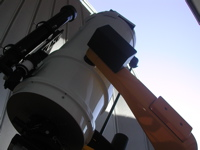# Astronomy Lab and Clay Telescope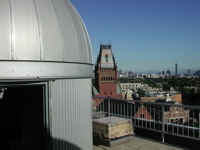Measurement of the Sun's Angular Diameter

printer version

The purpose of this experiement is to provide you with experience in making measurements, analyzing them, and understanding the results. The specific goal is to measure the Sun's angular diameter, as viewed from the Earth, by two different methods and to compare the results from the two methods to determine whether or not they are consistent.

 Method 1 - Pinhole Experiement Procedure. Have sunlight shine through the pinhole board with the emerging light forming an image of the Sun on a piece of paper taped to the floor about 3 m from the pinhole (Figure 1). Make pencil marks on the paper at opposite ends of the diameter of a Sun's image. With a ruler, measure the distance between these two marks. Also, carefully measure the distance between the pinhole and the image of the Sun on the paper, using a tape measure or meter stick. Repeat this pair of measurements, three to five times, recording all of your pairs of measurements. Analysis. From your data, form the ratio of the diameter of the Sun's image to the distance of this image from the pinhole. This ratio provides a good estimate in radians (2π radians equals 360 degrees) of the angular diameter of the Sun as viewed from the Earth. Explain why. [Hint: For small angles, θ (<< 1 radians), the ratios d/D and r/R (see Figure 2) are equal.] Also, estimate the uncertainty in your determination of this angular diameter.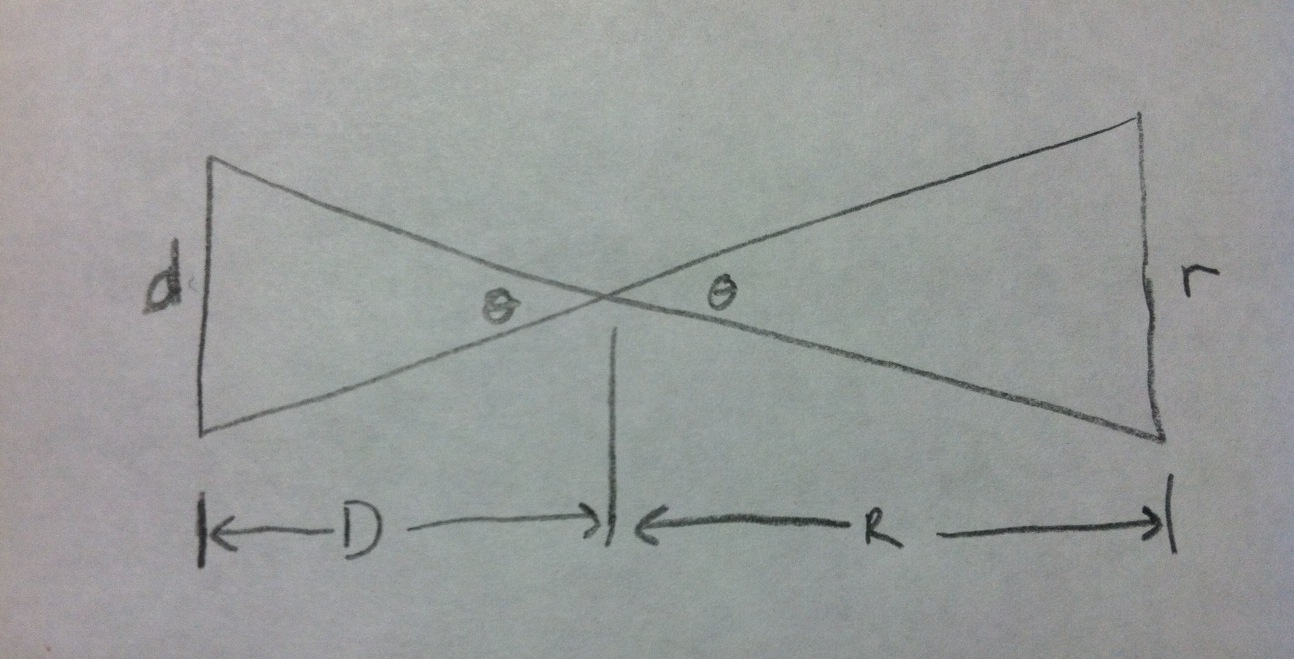Figure 2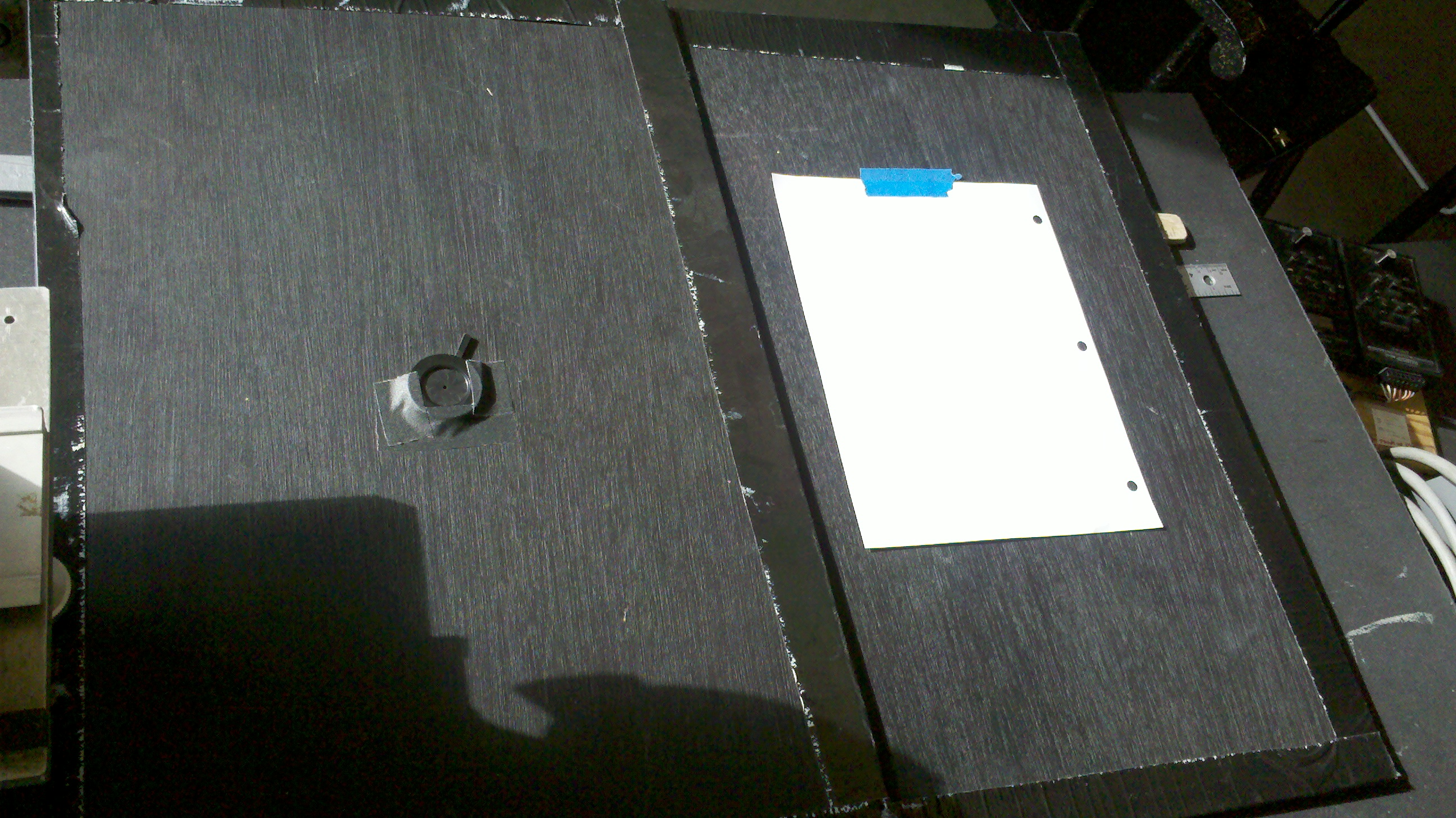Figure 1: Above are the pinhole boards that will be used to take measurements. The board on the left has an adjustable pinhole and will be used to direct the sunlight onto the sheet of paper on the second board.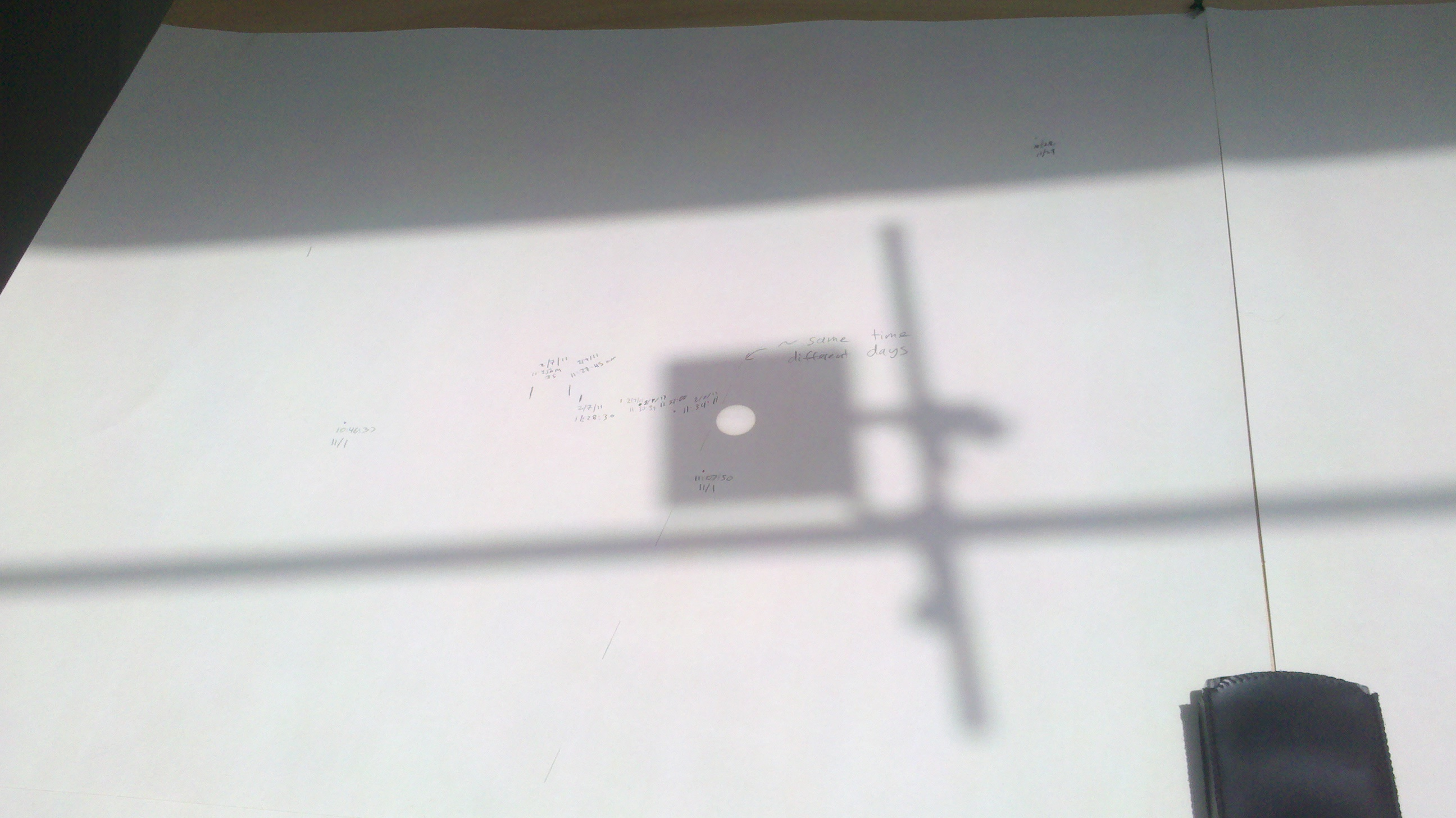Figure 3: The above image shows what the sunlight shone through the lens projects onto the easel. The shadow is the lens apparatus and the bright circle is the projection of the sun. Method 2 - Timing Experiment Procedure. There is a lens attached to the lab window that shines an image of the Sun on the lab easel (Figure 3). Students should form groups of two for this experiment. One person should mark the ends of the horizontal diameter of the Sun at the SAME TIME as a second person notes the exact time (including seconds), using the radio controlled clock provided. Measure the time interval for the Sun's image to move a distance equal to the Sun's diameter, as projected on the easel. That is, measure the time interval taken for the image to move completely through its own diameter. Repeat the measurement at least three times, as the available time allows. Record all of your data. Analysis. Knowing that the Earth makes a complete rotation in about 86,400 seconds, calculate the angular diameter of the Sun in radians as the ratio of the average of the times it took for the Sun to move through this diameter divided by 86,400 and multiplied by 2π. Your report should include a derivation/explanation as to why this formula gives a correct representation of the Sun's angular diameter. Estimate the error in your result. Also, estimate the effect on your result of having ignored the motion of the Earth in its orbit about the Sun.

Conclusion. Compare your result and its accompanying estimated error from Method 1 with those from Method 2. Discuss possible reasons for any differences that you obtained.